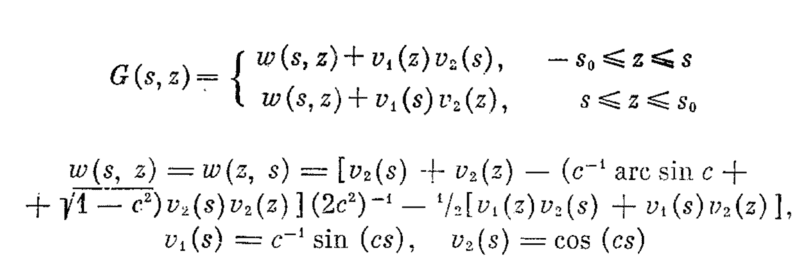# Build Green's function of an integro-differential operator

Gold Member

## Homework Statement

For a given ##a##, define $$B[u(x)] = u''(s) + \cos^2(a) u(s) - \frac{1}{2s_0}\int_{-s_0}^{s_0}(u''(s) + \cos^2(a) u(s) )\, ds,\\ s_0 = \frac{1}{\cos(a)}\arcsin(\cos a)$$

subject to boundary conditions

$$u'(s_0) + \cot (a) \cos (a) u(s_0) = 0\\ -u'(-s_0) + \cot (a) \cos (a) u(-s_0) = 0.$$

What is the Green's function associated with ##B[u(s)] = f## and it's boundary conditions?

## Homework Equations

Nothing comes to mind.

## The Attempt at a Solution

I attempted variation of parameters without considering the integral portion (which must be considered), but that technique failed: the two solutions I got from solving the ODE at ##-s_0## and ##+s_0## were linearly dependent, so their Wronskian was zero, and thus variation of parameters failed.

Does anyone have any idea how to solve this?

Gold Member
Does anyone know how to find Green's functions from this equation form? Anything helps (this is not HW, I just posted here since it seemed appropriate). I know there's a method for solving this problem, because I know the solution, which has a weird form.

Happy to share with anyone who is interested and can help.

Last edited:
The integral in your differential equation (differential operator) evaluates to a constant. This is not the form of a differential operator which can be solved by the Green's function technique. Are you sure you wrote the equation correctly? It could work if the integral was multiplied by u or u'. Also, your integral appears to be incorrect. If it is written instead: $$I=\frac {u(s)}{2s_0} \int_{-s_0}^{s_0}\left (u''(s)+\cos ^2 (a)u'(s) \right ) ds \\ u"(s)ds = du'(s) \\ u'(s)ds = du(s) \\I= \left. \frac {u(s)}{2s_0} \left (u'(s) \right |_{-s_0}^{s_0} + \left. \cos ^2 (a) u(s) \right |_{-s_0}^{s_0} \right )$$
$$=\frac {u(s)}{2s_0} \left ( u'(s_0) - u'(-s_0) + \cos ^2(a) (u(s_0) - u(-s_0)) \right )$$
I multiplied the integral by ##u(s)## (harmonic component) but ##u'(s)## (real exponential component) would also work. Please see http://www.damtp.cam.ac.uk/user/dbs26/1BMethods/GreensODE.pdf for an explanation on how to build Green's functions.

Last edited:
Gold Member
The integral in your differential equation (differential operator) evaluates to a constant. This is not the form of a differential operator which can be solved by the Green's function technique. Are you sure you wrote the equation correctly?
It is of the form because I have the Green's function, I just don't know how to get there. I wrote the equation correctly, sadly. I've copy-pasted it for your consideration.

Please see http://www.damtp.cam.ac.uk/user/dbs26/1BMethods/GreensODE.pdf for an explanation on how to build Green's functions.
Yea I know how to build Green's functions, but in this case the standard variation of parameters approach fails, and fails big time.

I appreciate your interest, but do you (or anyone else) know how to find the Green's function for the problem posted? For completeness, it is attached. It should also be noted that ##c## is defined as ##\cos(a)##. Also, ##v_1## and ##v_2## are solutions to $$v'' + \cos^2 (a) v = 0\\ v_1(0) = 0,\,\, v_1'(0) = 1\\ v_2(0) = 1,\,\, v_2'(0) = 0$$